3-reel slot machines

1. Case A – the same number of stops and symbol distribution on the reels

1.7  Event– Any combination of at least one of three specific symbols

The probability ofis, where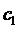,, and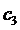are the distributions of the three symbols on a reel.

For the numerical probabilities, we can use the table and the results of section 1.1 (event- A specific symbol three times) for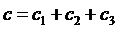.

Example:

Find the probability of any combination of seven-type symbols (assume for example that there are red, blue, and black sevens) occurring on a payline of a 3-reel slot machine with 48 stops on each reel, having 2 red, 2 blue and 3 black sevens on each reel.

Take for instance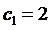,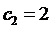, and(for any permutation of these values on the denotations, we will reach the same result, as the probability formula is symmetric in these three variables). We take c = 2 + 2 + 3 = 7 and look in the table of section 1.1 at the intersection of row t = 48 with column c = 7, where we find the probability P = 0.00310149 = 0.310149%.